Orginal Article

# 复杂加载条件下的砂土本构模型

(Foundation Engineering Research Institute,China Academy of Building Research, Beijing 100013,China )

Abstract

Abstract The test shows that stress-strain relationship of saturated sand has significant dependence on density and confining pressure. The above two factors can not be ignored to describe the deformation behavior of sand under static load conditions. In addition, saturated sand also exhibits obvious stress-induced anisotropy and phase transformation behaviors under complex loading, such as cyclic loading conditions. The distance $R$ between the current stress state and its corresponding point in critical state line (CSL) can be treated as a state parameter is introduced into the proposed model to reflect the density and confining pressure dependent behaviors based on the assumption that there is a unique CSL in $e$--$p$ space. The influence principle to stress-strain relationship under monotonic loading condition due to density and confining pressure is accurately described by using unified hardening parameter introduced by phase changing stress ratio and peak stress ratio expressed by exponential functions of state parameter. The shear volume compression, dilatancy, strain softening and hardening are all described for sand. By using non-associated flow rule, a water drop shape yield surface and an ellipse shape plastic potential surface are adopted in $p$--$q$ space. The liquefaction phenomenon under monotonic loading condition are also be described. To reflect the accumulation of plastic volume strain and hysteresis loops of deviatoric plastic strain under cyclic loading condition, the state parameter $R$ can be expressed as stress ratio parameter and the rotational hardening part can be adopted to describe the stress-induced anisotropy are introduced into the hardening parameter. The attenuation of shear modulus, stiffness weaken and strength decreasing behaviors are described effectively by using the proposed model. The cyclic mobility phenomenon is predicted under undrained cyclic loading conditions. The effectiveness and applicability of the proposed constitutive model is verified by the comparison of a series of simulation and test results.

Keywords： density and confining pressure dependent behaviors ; liquefaction ; cyclic mobility ; constitutive model ; cyclic loading

0

Wan Zheng, Meng Da. A CONSTITUTIVE MODEL FOR SAND UNDER COMPLEX LOADING CONDITIONS[J]. Acta Mechanica Sinica, 2018, 50(4): 929-948 https://doi.org/10.6052/0459-1879-18-047

## 1 $e$-- $p$空间中的压缩线及状态线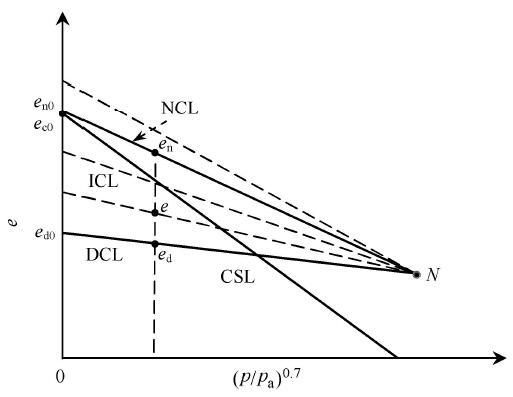Fig. 1   Series of compression lines and critical state line in $e$ versus $(p/pa)ζ$ space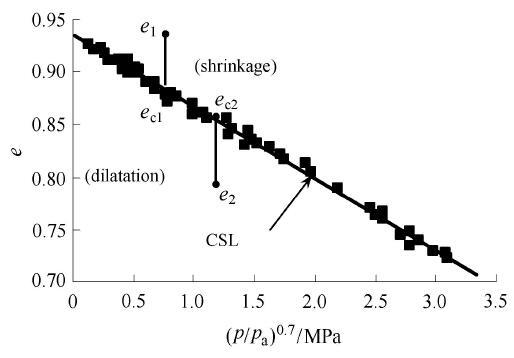Fig. 2   Critical state line of Toyoura sand in $e$ versus ($p/pa)ζ$ space

## 2 本构模型

### 2.1 $p$--$q$空间中的屈服面与塑性势面参数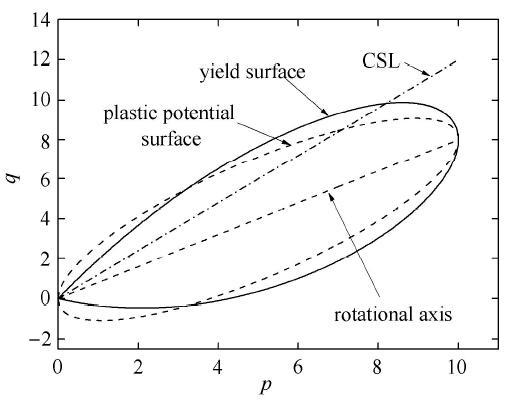Fig. 3   Yielding surface and Plastic potential surface in $p$ versus $q$ space

$Mc=Maexp(-mR),monotonicMa,cyclic(10)$

$Mf=Mexp(nR),-1.5cmmonotonic6M2123-MR1+M2123-MR-M2123-MR,-1.5cmcyclic(11)$

$Mp=Mexp(-mR),monotonicM,cyclic(12)$

$Mn=Mexp(nR1),monotonicM,cyclic(14)$

$R1=ec-en(15)$

$A=1.5ηη*ζPmb-βpaζp1+η*2Ma2-χη*2ζ-1⋅$

$3η̂ijβij-2Ma2Ma2-χη*22(17)$

### 2.2 状态参量 $χ$的确定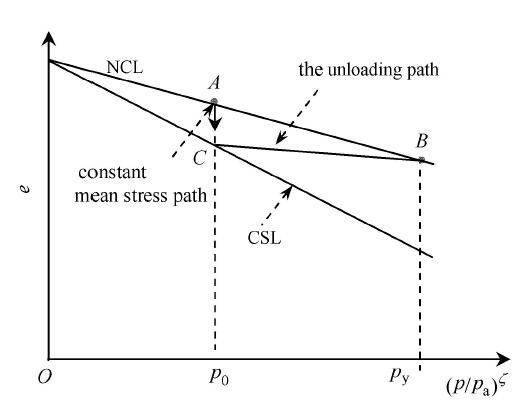Fig. 4   Isotropic loading and unloading curves of sand in $e$--$(p/pa)ζ$ space

### 2.3 硬化参数确定

2.3.1 等向硬化参数分析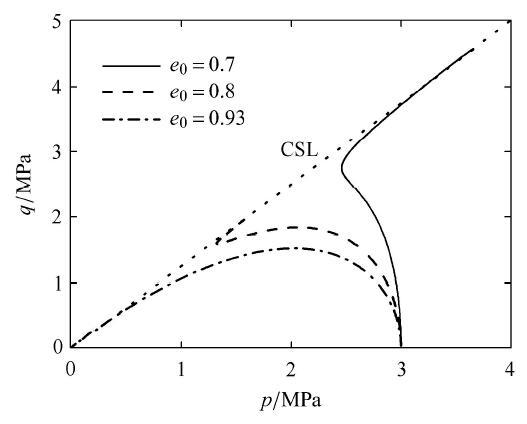Fig. 5   Three typical stress paths of sand under undrained condition in $p$--$q$ space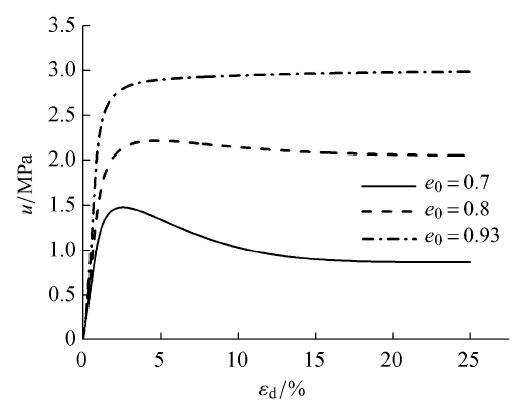Fig. 6   Three typical pore pressure curves with different initial density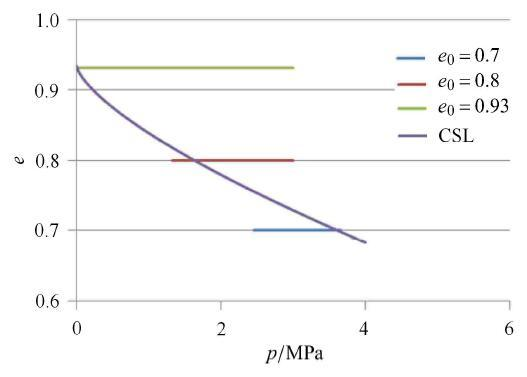Fig. 7   Three typical stress paths of sand under undrained condition in $e$--$p$ space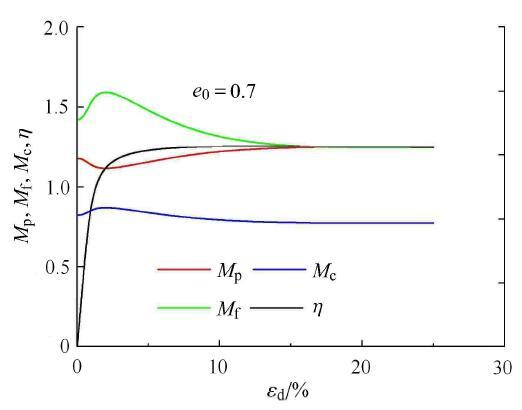Fig. 8   Changing process of four kinds of stress ratios with void ratio equals 0.7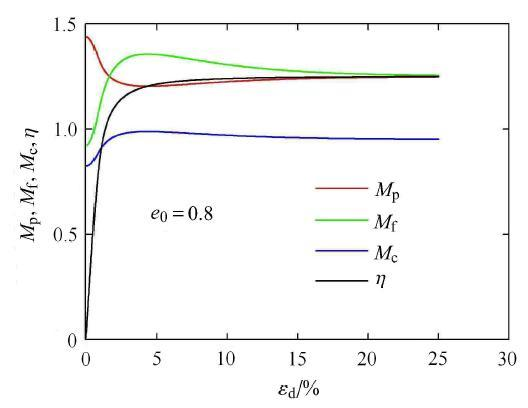Fig. 9   Changing process of four kinds of stress ratios with void ratio equals 0.8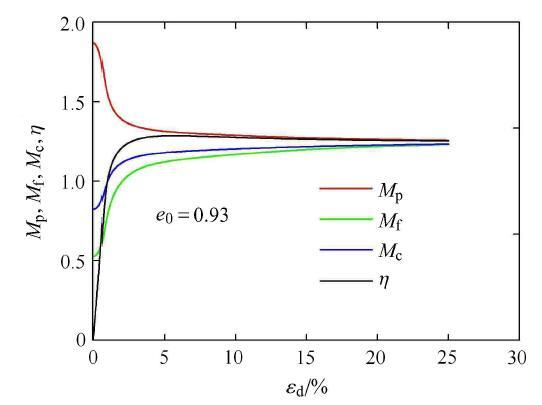Fig. 10   Changing process of four kinds of stress ratios with void ratio equals 0.93

(1)当砂土 属于密砂时,则当应力比$η<Mp<Mf$时,由于$dεvp>0$, 则$dH1>0$,属于应变硬化阶段,当$Mp<η<Mf$时,越过变相应力比,此时由于$dεvp<0$$dH1>0$,属于应变硬化阶段,当$Mp=η=Mf=M$时,$dεvp=0$,则最终达到临界状态.

(2)当砂土 属于松砂时,则当应力比 $η时,由于 $dεvp>0$, 则 $dH1>0$,属于应变硬化阶段,当 $Mf<η时,应力比未达到变相应力比,由于 $dεvp>0$,则 $dH1<0$,属于应变软化阶段,当 $Mp=η=Mf=M$时, $dεvp=0$,则最终达到临界状态;当 $η时且此时 $dσij=0$,则达到液化状态.

(3)考察当进行等方向压缩应力路径时,则由于 $η=0$, $dH1=Mf4Mn4dεvp$,则当初始密度位于NCL线上时,则由 $Mn$表达式可知, $Mn=Mf$,此时, $dH1=dεvp$,退化到修正剑桥模型,当初始密度在NCL线以上,属于松砂状态时,则由于 $Mf,此时导致较大的塑性体变增量产生,反之,当属于中密砂时,则由于 $Mf>Mn$,此时导致较小的塑性体变增量产生,上述规律与等方向压缩时的体变规律相符.

2.3.2 旋转硬化参数分析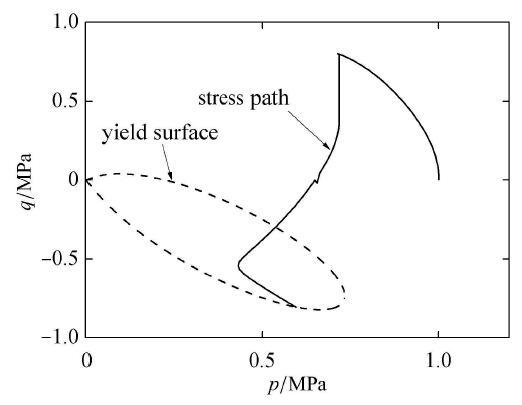Fig. 11   Figure 1 of process of stress path for cyclic mobility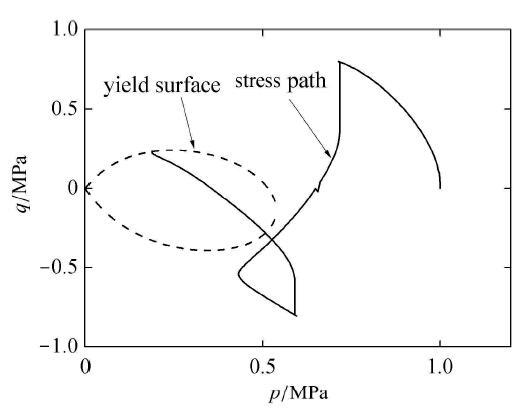Fig. 12   Figure 2 of process of stress path for cyclic mobility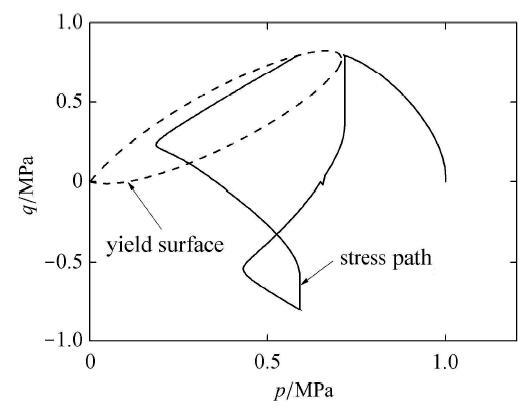Fig. 13   Figure 3 of process of stress path for cyclic mobility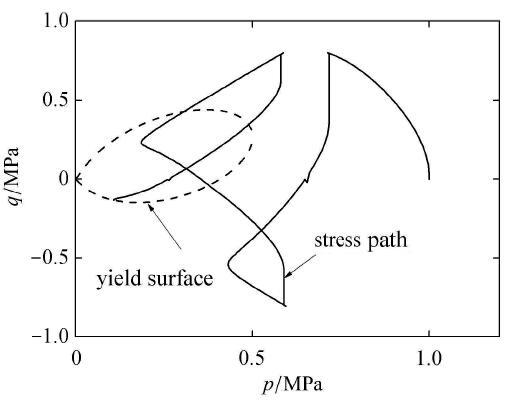Fig. 14   Figure 4 of process of stress path for cyclic mobility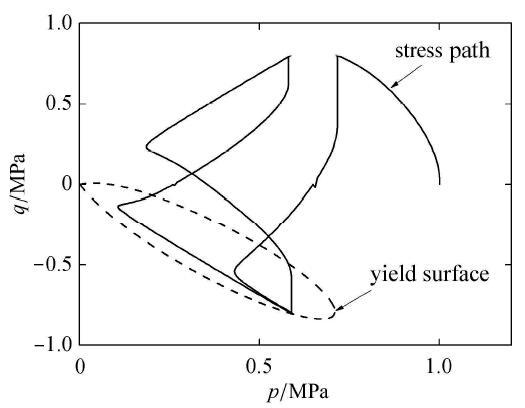Fig. 15   Figure 5 of process of stress path for cyclic mobility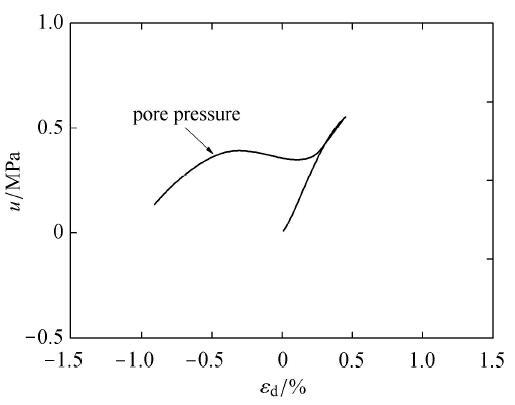Fig. 16   Figure 1 of process of pore water pressure for cyclic mobility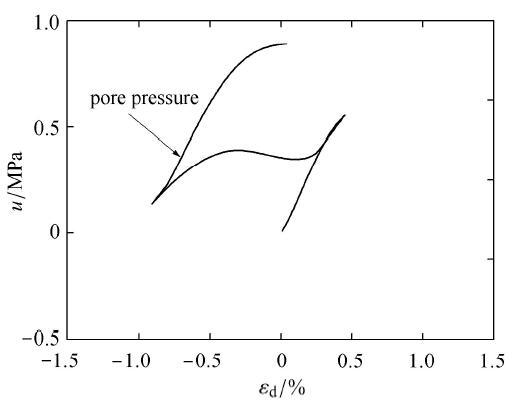Fig. 17   Figure 2 of process of pore water pressure for cyclic mobility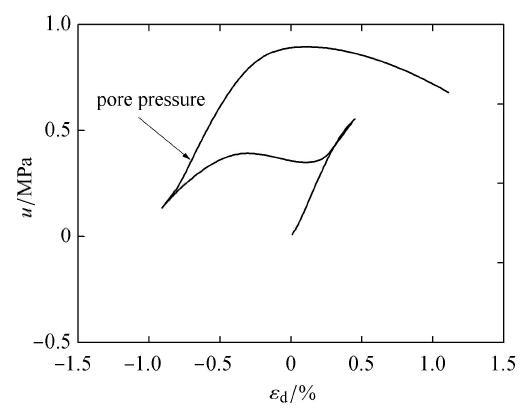Fig. 18   Figure 3 of process of pore water pressure for cyclic mobility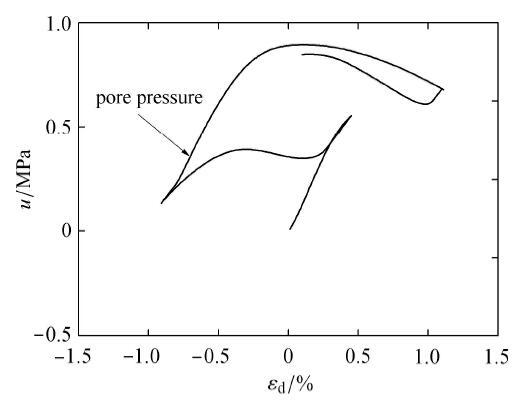Fig. 19   Figure 4 of process of pore water pressure for cyclic mobility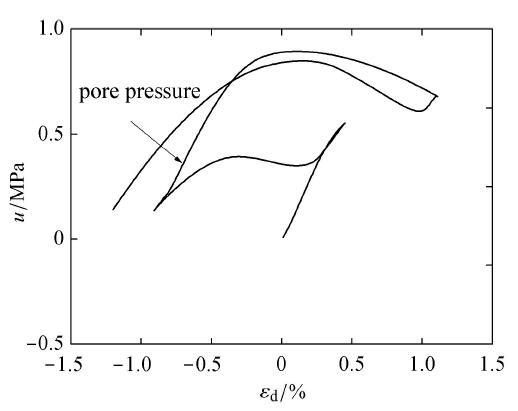Fig. 20   Figure 5 of process of pore water pressure for cyclic mobility

Seed曾把在循环加载后第一次发生液化时候的状态称之为“初始液化”,循环加载后也会产生往返活动性现象. 由于状态 参量 $χ$的影响,所建议的模型分别能够模拟初始液化现象以及往返活动性现象.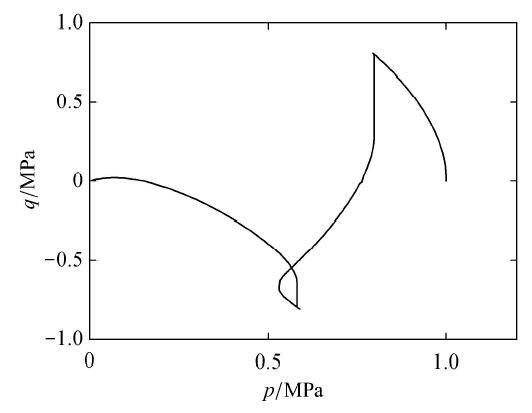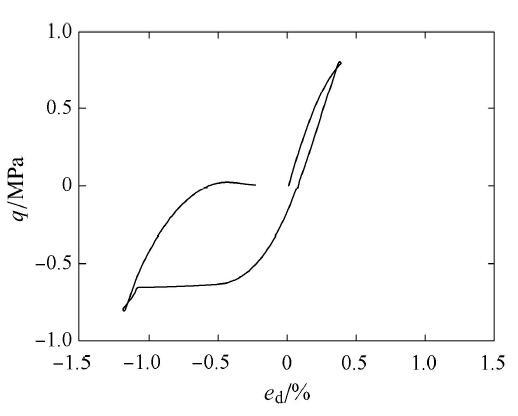Fig. 22   Stress strain relationship of liquefaction under undrained cyclic loading condition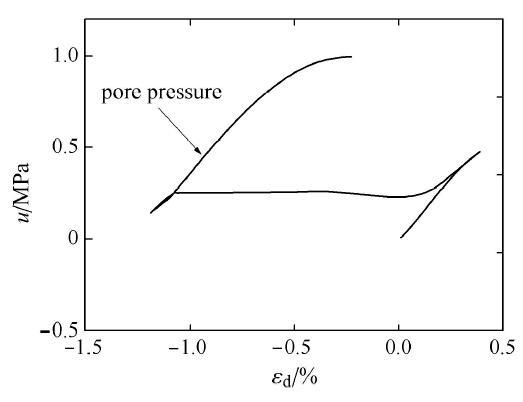Fig. 23   Figure of process of pore water pressure for cyclic mobility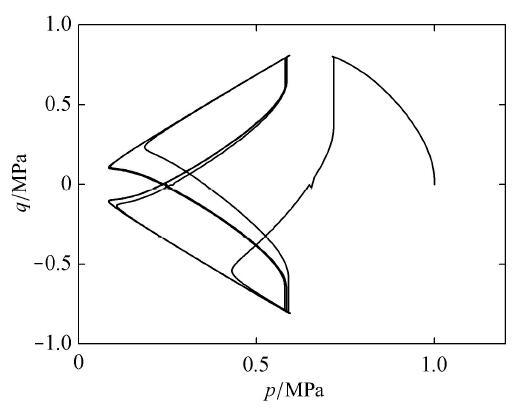Fig. 24   Typical stress path of cyclic mobility phenomena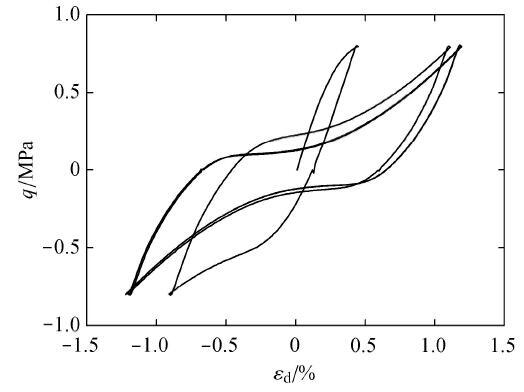Fig. 25   Typical stress strain relationship of cyclic mobility phenomena

## 3 本构方程

### 3.1 弹塑性本构方程

$Ω=Mp4Mn4Mf4-η4Mp4-η4(37)$

$A=1.5ηη*ζPmb-βpaζp1+η*2Ma2-χη*2ζ-1⋅$

$3η̂ijβij-2Ma2Ma2-χη*22(38)$

$∂f∂σijDijkledεkl-λ∂f∂σijDijkle∂g∂σkl+∂f∂βijdβij-Mp4Mn4Mf4-η4Mp4-η4dεvp䆃$

$∂f∂σij=ζcppaζp1+η*2Ma2-χη*2ζ-1⋅131+η*2Ma2-χη*2δij+Ma23η̂ij-ηklη̂klδijMa2-χη*22$

(44)

$∂g∂σij=ζcppaζp1+η*2Mc2ζ-1⋅131+η*2Mc2δij+3η̂ij-ηklη̂klδijMc2(45)$

$∂f∂βijdβij=3λmb-βη*2ζ2pMcpMc2pa2ζMa2-χη*22⋅p1+η*2Ma2-χη*2ζ-1p1+η*2Mc2ζ-1⋅3η̂klβkl-2Ma2(46)$

$dσij=Dijkldεkl(47)$

$Dijkl=Dijkle-Dijmne∂g∂σmn∂f∂σstDstkle/X(48)$

$Dijkl=Lδijδkl+Gδikδjl+δilδjk-$

$L∂g∂σmmδij+2G∂g∂σijL∂f∂σnnδkl+2G∂f∂σkl/X$

(49)

$X=L∂f∂σii∂g∂σ̃kk+2G∂f∂σij∂g∂σij+T1+T2(50)$

$T1=Mp4Mn4Mf4-η4Mp4-η4∂g∂σijδij(51)$

$T2=ηM-1∂f∂βijdβij(52)$

## 4 模型预测

### 4.1 单调加载下模型预测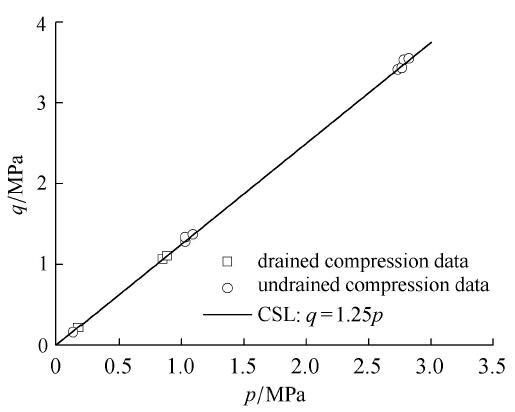Fig. 26   Calibration of M for slope of CSL in $p$--$q$ space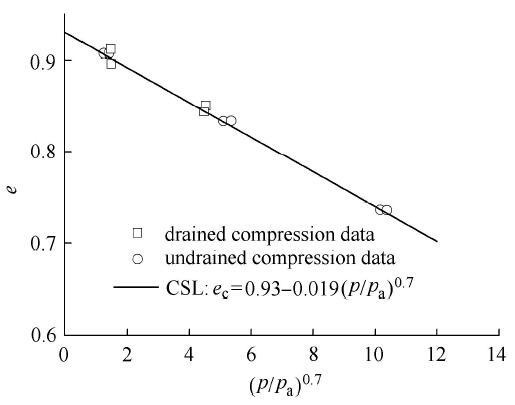Fig. 27   Calibration of CSL in $e$--$p$ space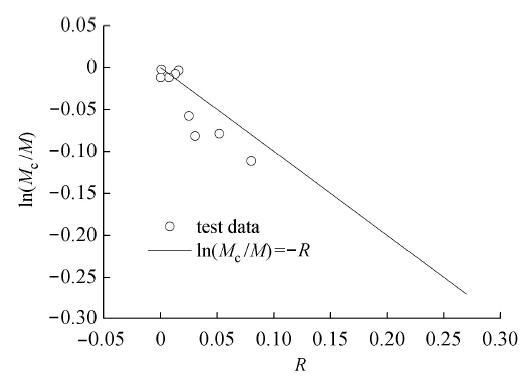Fig. 28   Calibration of phase transformation stress ratio parameter $m$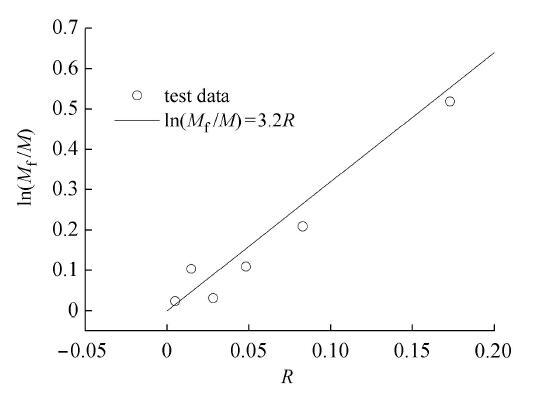Fig. 29   Calibration of peak stress ratio parameter $n$

$mb$为转轴界限值应力比参数,一般在0 $~M$之间,对于应力诱导各向异性显著材料,则取值较大,可用于反映应力历史对于当前模量及强度的影响. $α$为反映循环 加载下应力路径对于当前体变衰减的影响系数. 当应力路径对当前体变影响显著,则取较大值,反之则取较小值. 其范围在0 $~$1之间.

Table 1   Parameter of Toyoura sand

$M$$ct$$ce$$en0$$mb$$α$$ζ$$cc$$ν$$m$$n$
.250.010.003 20.93010.70.0190.313.2

Verdugo和Ishihara曾针对Toyoura饱和砂土进行了一系列三轴剪切加载测试. 加载方式包括不排水常规三轴剪切以及排水常规三轴剪切. 图30为初始孔隙比为0.735时的不排水应力路径测试以及预测对比图. 在较大初始密度下,分别对应有4种初始球应力作为初始压密状态,分别为0.1, 1.0, 2.0, 3.0 MPa. 在同样密度下,随着围压的增大,砂土由剪缩到剪胀的过渡应力比即变相应力比逐渐增大,高围压下密砂表现出类似于松砂在低围压下的路径特点.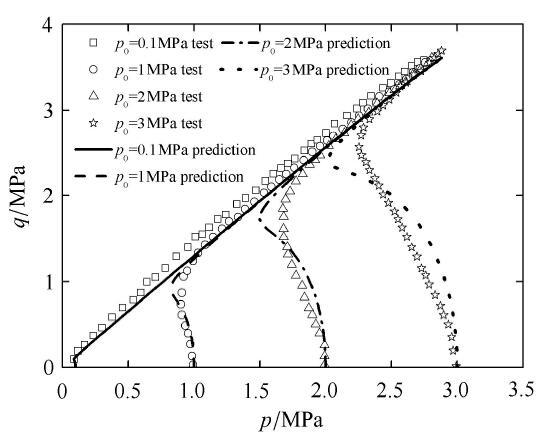Fig. 30   Comparison between prediction and test results for stress paths under undrained triaxial compression condition with void ratio equals 0.735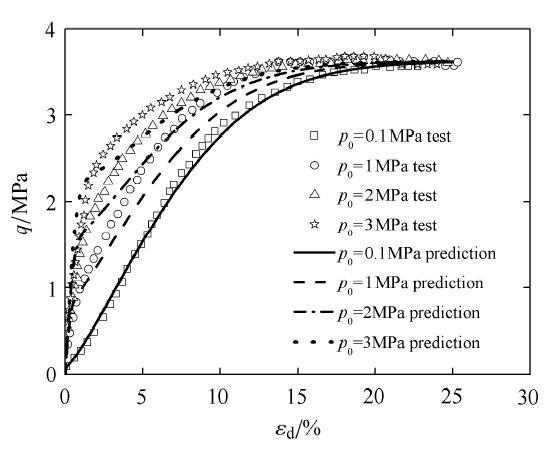Fig. 31   Comparison between prediction and test results for stress-strain relationship under undrained triaxial compression condition with void ratio equals 0.735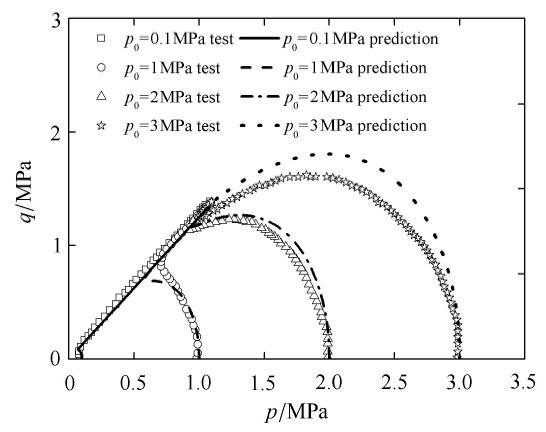Fig. 32   Comparison between prediction and test results for stress paths under undrained triaxial compression condition with void ratio equals 0.833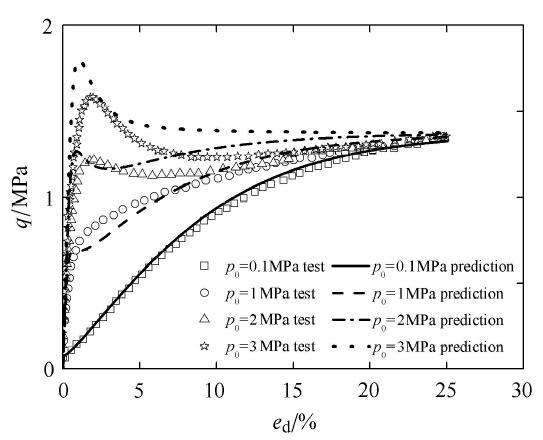Fig. 33   Comparison between prediction and test results for stress-strain relationship under undrained triaxial compression condition with void ratio equals 0.833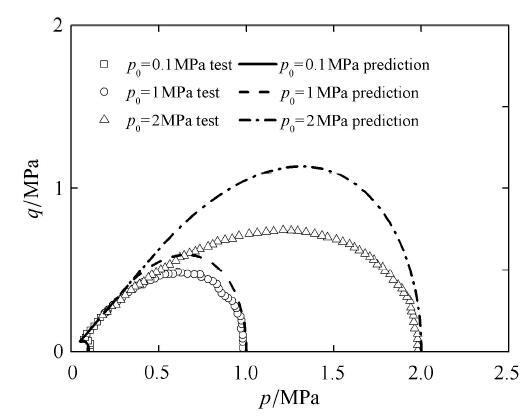Fig. 34   Comparison between prediction and test results for stress paths under undrained triaxial compression condition with void ratio equals 0.907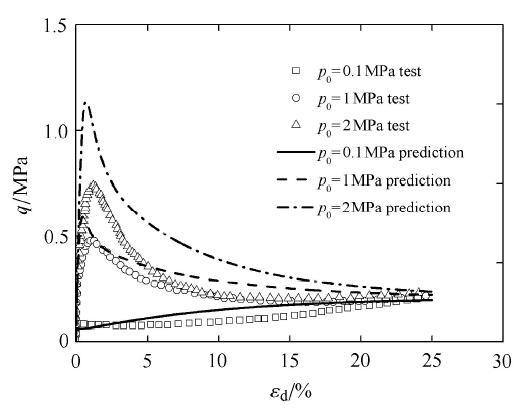Fig. 35   Comparison between prediction and test results for stress-strain relationship under undrained triaxial compression condition with void ratio equals 0.907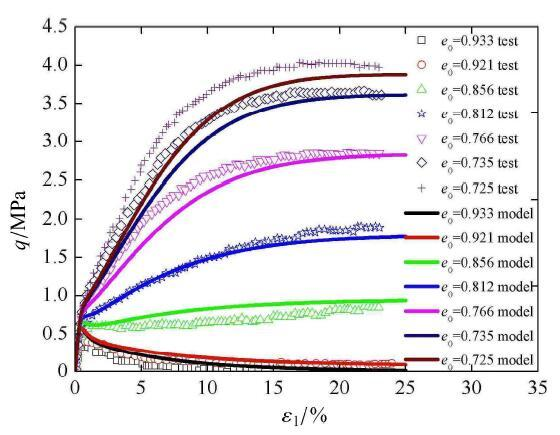Fig. 36   Comparison between prediction and test results for stress-strain relationship under undrained triaxial compression condition with various initial value of void ratio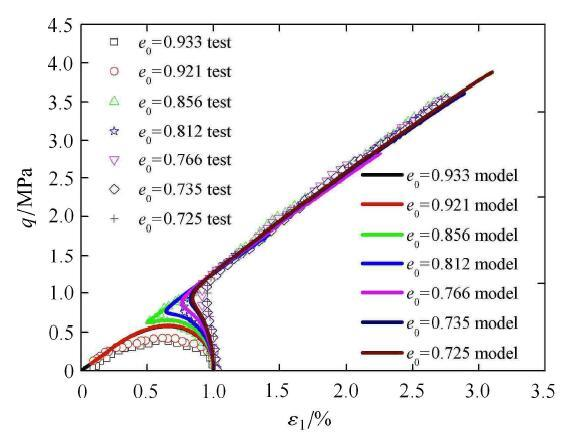Fig. 37   Comparison between prediction and test results for stress paths under undrained triaxial compression condition with various initial value of void ratio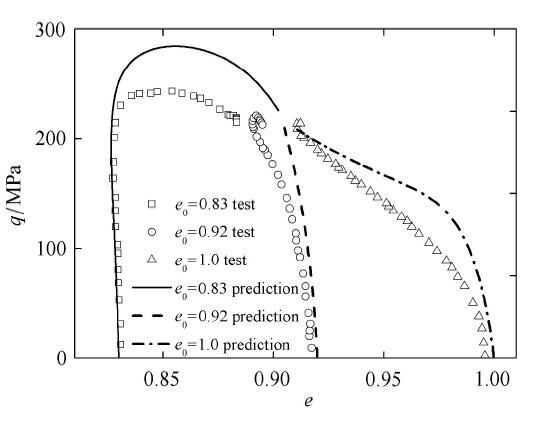Fig. 38   Comparison between prediction and test results for generalized deviatoric stress versus void ratio under drained triaxial compression condition with initial value of $p0$ equals 0.1 MPa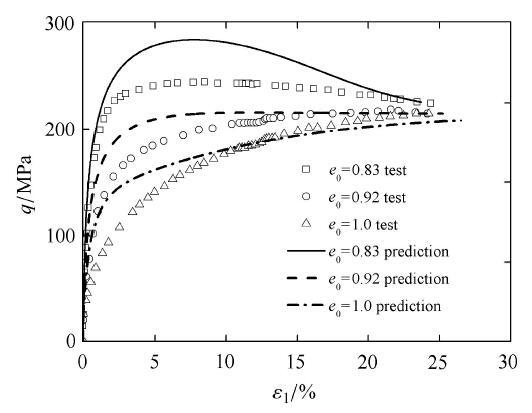Fig. 39   Comparison between prediction and test results for generalized deviatoric stress versus the principal strain under drained triaxial compression condition with initial value of $p0$ equals 0.1 MPa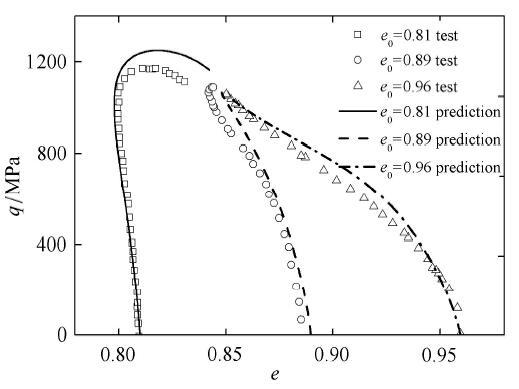Fig. 40   Comparison between prediction and test results for generalized deviatoric stress versus void ratio under drained triaxial compression condition with initial value of $p0$ equals 0.5 MPa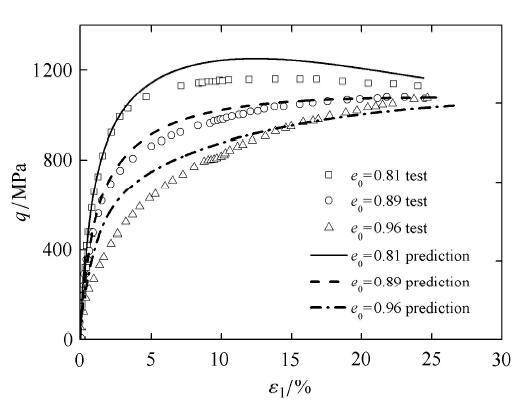Fig. 41   Comparison between prediction and test results for generalized deviatoric stress versus the principal strain under drained triaxial compression condition with initial value of $p0$ equals 0.5 MPa

Table 2   Parameter of Sacramento sand

$M$$ct$$ce$$en0$$mb$$α$$ζ$$cc$$ν$$m$$n$
.250.010.003 20.94010.70.0190.313.3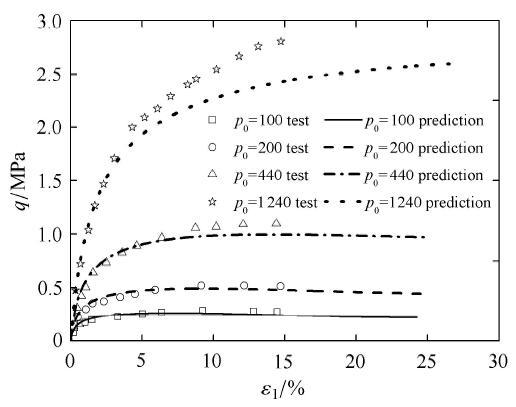Fig. 42   Comparison between prediction and test results for generalized deviatoric stress versus the principal strain under drained triaxial compression condition of Sacramento river loose sand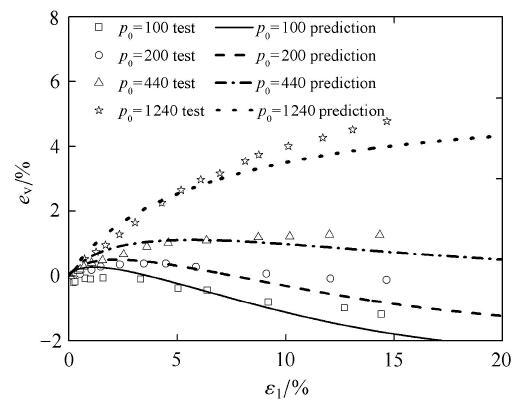Fig. 43   Comparison between prediction and test results for volumetric strain versus the principal strain under drained triaxial compression condition of Sacramento river loose sand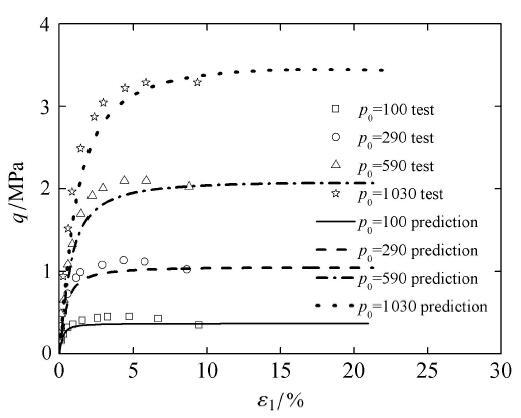Fig. 44   Comparison between prediction and test results for generalized deviatoric stress versus the principal strain under drained triaxial compression condition of Sacramento river dense sand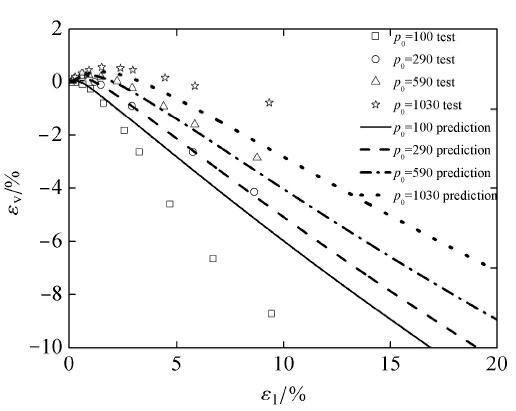Fig. 45   Comparison between prediction and test results for volumetric strain versus the principal strain under drained triaxial compression condition of Sacramento river dense sand

### 4.2 循环加载下模型预测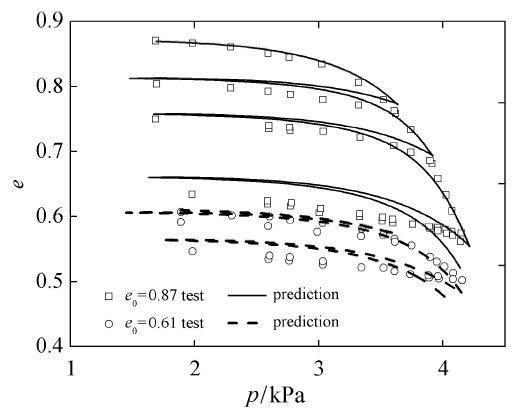Fig. 46   Comparison between prediction and test results for void ratio versus the mean stress under isotropic compression condition of Sacramento river sand

Table 3   Parameter of Niigata sand

$M$$ct$$ce$$en0$$mb$$α$$ζ$$cc$$ν$$m$$n$
.30.0350.002 50.881.250.10.70.0560.313.3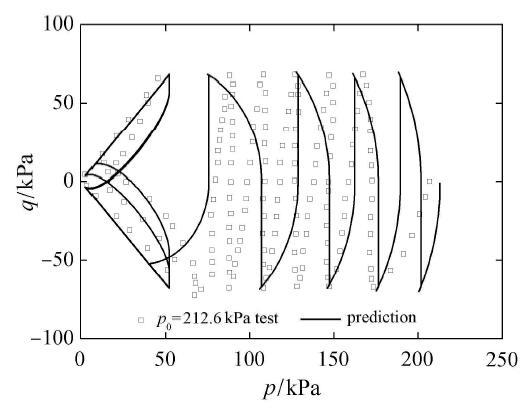Fig. 47   Comparison between prediction and test results for stress path under two way undrained cyclic loading condition of Niigata sand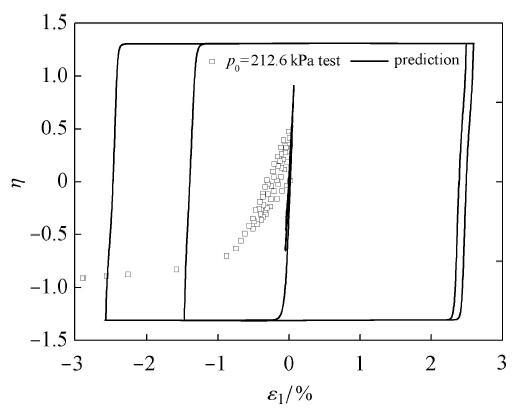Fig. 48   Comparison between prediction and test results for stress strain relationship under two way undrained cyclic loading condition of Niigata sand

Table 4   Parameter of Toyoura sand

$M$$ct$$ce$$en0$$mb$$α$$ζ$$cc$$ν$$m$$n$
.050.050.002 10.951.00.30.40.10.313.3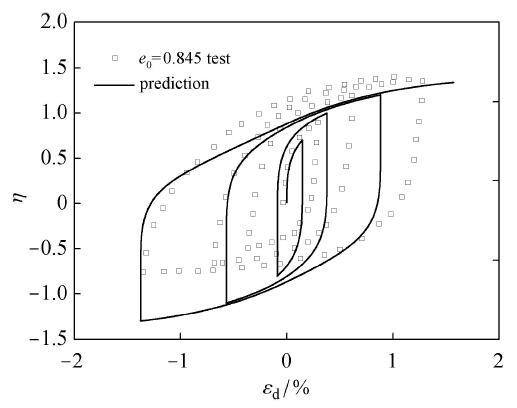Fig. 49   Comparison between prediction and test results for stress strain relationship under drained compression with constant $p$=98.1kPa loading condition of Toyoura loose sand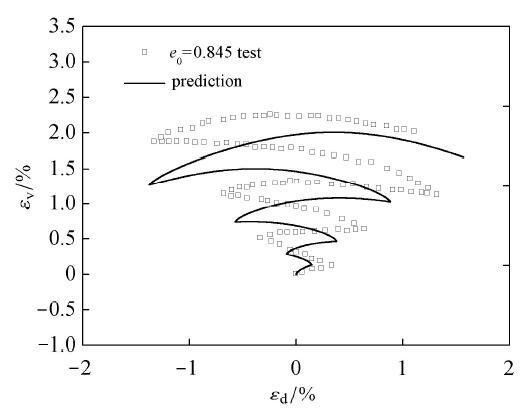Fig. 50   Comparison between prediction and test results for volumetric strain versus generalized deviatoric strain under drained compression with constant $p=98.1$ kPa loading condition of Toyoura loose sand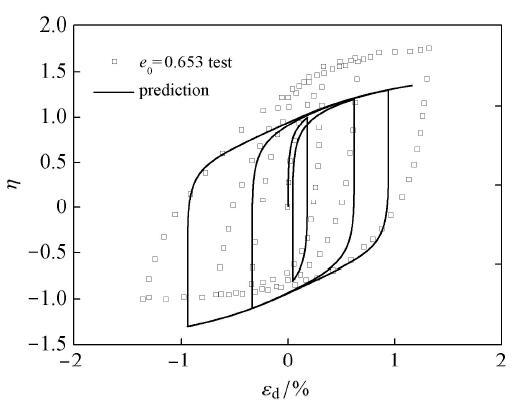Fig. 51   Comparison between prediction and test results for stress strain relationship under drained compression with constant $p$=98.1kPa loading condition of Toyoura dense sand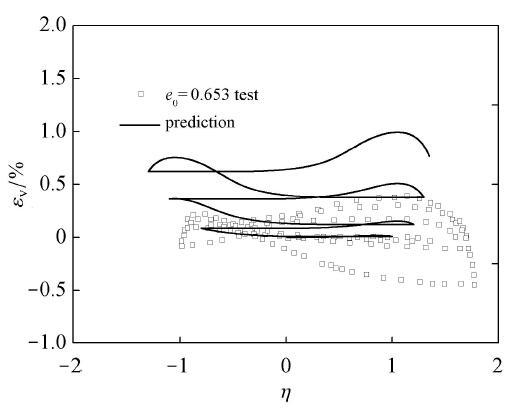Fig. 52   Comparison between prediction and test results for volumetric strain versus generalized deviatoric strain under drained compression with constant $p=98.1$ kPa loading condition of Toyoura dense sand

## 5 结 论

(1) 模型通过引入状态参量$R$来表示相同球应力下临界状态线上的孔隙比与当前孔隙比之差,并将变相应力比、潜在强度比表示为状态参量的指数函数,该指数函数能反映密砂变相应力比小、潜在应力比大,松砂变相应力比大而潜在强度比小等特征,利用过渡应力比来修正统一硬化参数,使得硬化参数能同时考虑剪切体缩、体胀规律特性以及等方向压缩的体缩特性.

(2) 引入修正屈服面形状的状态参量 $χ$,通过该参数与硬化参数配合使用能反映松砂应变软化力学特性,如静态以及循环加载液化现象.

(3) 在硬化参数中引入了考虑应力诱导各向异性的旋转硬化参量部分,与各向同性硬化部分共同作用. 由于屈服面与塑性势面均引入了能反映循环加载作用特性的转轴,塑性偏应变也对屈服面的硬化过程贡献作用. 在不排水往复循环作用下,能反映塑性体应变的循环累积作用,能反映塑性偏应变的往复滞回特点,如往返活动性现象. 同时对于松砂以及密砂的循环加载作用响应结果能较好的描述.

The authors have declared that no competing interests exist.

## 参考文献 原文顺序 文献年度倒序 文中引用次数倒序 被引期刊影响因子〈〉